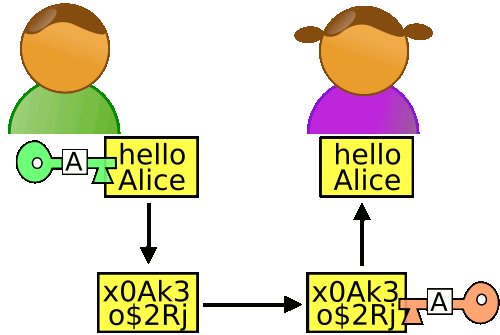# [转]RSA算法原理（二）

http://www.ruanyifeng.com/blog/2013/07/rsa_algorithm_part_two.htmln = 61×53 = 3233

n的长度就是密钥长度。3233写成二进制是110010100001，一共有12位，所以这个密钥就是12位。实际应用中，RSA密钥一般是1024位，重要场合则为2048位。

φ(n) = (p-1)(q-1)

ed ≡ 1 (mod φ(n))

ed – 1 = kφ(n)

ex + φ(n)y = 1

17x + 3120y = 1

p
q
n
φ(n)
e
d

（1）ed≡1 (mod φ(n))。只有知道e和φ(n)，才能算出d。

（2）φ(n)=(p-1)(q-1)。只有知道p和q，才能算出φ(n)。

（3）n=pq。只有将n因数分解，才能算出p和q。

”对极大整数做因数分解的难度决定了RSA算法的可靠性。换言之，对一极大整数做因数分解愈困难，RSA算法愈可靠。

12301866845301177551304949
58384962720772853569595334
79219732245215172640050726
36575187452021997864693899
56474942774063845925192557
32630345373154826850791702
61221429134616704292143116
02221240479274737794080665
351419597459856902143413

33478071698956898786044169
84821269081770479498371376
85689124313889828837938780
02287614711652531743087737
814467999489
×
36746043666799590428244633
79962795263227915816434308
76426760322838157396665112
79233373417143396810270092
798736308917

（1）加密要用公钥 (n,e)

me ≡ c (mod n)

6517 ≡ 2790 (mod 3233)

（2）解密要用私钥(n,d)

cd ≡ m (mod n)

27902753 ≡ 65 (mod 3233)

cd ≡ m (mod n)

ｍe ≡ c (mod n)

c = me – kn

(me – kn)d ≡ m (mod n)

med ≡ m (mod n)

ed ≡ 1 (mod φ(n))

ed = hφ(n)+1

mhφ(n)+1 ≡ m (mod n)

（1）m与n互质。

mφ(n) ≡ 1 (mod n)

(mφ(n))h × m ≡ m (mod n)

（2）m与n不是互质关系。

(kp)q-1 ≡ 1 (mod q)

[(kp)q-1]h(q-1) × kp ≡ kp (mod q)

(kp)ed ≡ kp (mod q)

(kp)ed = tq + kp

(kp)ed = t’pq + kp

med ≡ m (mod n)

（完）